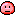## How to Expand Logarithms

There are a few different techniques for expanding logarithms, depending on the form of the expression. Here are some common techniques:

1. Using the logarithmic identity: log(b^a) = a log(b)
If you have a logarithm of a power, you can use this identity to expand it. For example:

log(2^3) = 3 log(2)

2. Using the logarithmic product rule: log(a * b) = log(a) + log(b)
If you have a logarithm of a product, you can use this rule to expand it. For example:

log(4 * 5) = log(4) + log(5)

3. Using the logarithmic quotient rule: log(a / b) = log(a) - log(b)
If you have a logarithm of a quotient, you can use this rule to expand it. For example:

log(8 / 2) = log(8) - log(2)

4. Using the change of base formula: log(b, x) = log(a, x) / log(a, b)
If you have a logarithm with a different base than 10 or e, you can use this formula to change the base, which can sometimes make it easier to expand. For example:

log(2, 8) = log(10, 8) / log(10, 2) = 3 / log(10, 2)

5. Combining techniques: Sometimes, you need to use more than one technique to fully expand a logarithm. For example:

log(2^3 * 4 / 10) = log(2^3) + log(4) - log(10) = 3 log(2) + log(4) - log(10)

PleaseSubject to conditionsComment